LLM

# Open Source Distributed Graph Computing Frameworks Comparison

## 1. Introduction

With the soaring of data in recent years, how to process and analyze data has become a hot topic. Data in the real world is quite large and complex. The new data generated by the interactions between those different data entities is most naturally presented in the form of graphs. The social network of WeChat is composed of nodes (users and public numbers) and edges (followers and likers), and the transaction network of Alibaba is composed of nodes (users and merchants) and edges (transactions and favorites).

Graph computing is precisely a technology that studies the relationship between data entities and portrays the data in the form of graphs. At present, several companies have applied graph computing technology to their business scenarios, such as Jingdong, Sougou, ICBC, etc. Graph computing technology has achieved much better results than traditional computing technology.

Meituan also has an urgent need to use graph computing in many scenarios such as rider matching networks, financial anti-fraud networks, and device risk detection networks, etc.

Graph computing technology can well solve the problem of offline analysis of full graph space data, but there are still some problems in the implementation. There are problems in graph computing such as data sparsity, power-law distribution of vertices, dynamic changes in active vertex sets, and high parallel communication overhead. Those make graph computing not have good parallel scalability, and the performance of poorly designed graph computing frameworks is even inferior to that of standalone machines.

To meet the super large-scale graph computing needs of Meituan, we need to pick up the best framework as the underlying engine of our graph computing platform. Considering our business scenarios, we make the following requirements for the graph computing framework selection.

1. Open-source project: Must have control over the source code to ensure data security and service availability.

2. Distributed architecture with good scalability.

3. Ability to deal with OLAP scenarios, outputting graph analysis results with high performance.

4. General-purpose graph computing system that can provide a variety of popular graph algorithms and can be easily customized to develop new algorithms for a variety of business application scenarios.

After having done a lot of research on graph computing frameworks, we list some representative graph computing frameworks as follows.

• Neo4j-APOC: Based on the graph database, it supports basic graph algorithms, and the distributed version is not open-source.

• Pregel: Proposed by Google in 2009, is the pioneer of the graph computing model, much subsequent work is influenced by its ideas, not open-source.

• Giraph: Facebook's open-source implementation based on Pregel's ideas.

• Gemini: Implemented by Tsinghua University based on Pregel's ideas. Free trials are provided. The commercial version is not open-source.

• KnightKing: A graph computing framework specially designed for the random walker algorithm.

• GraphX: A graph computing framework implemented based on Spark by Apache Foundation. The community is active.

• GraphLab (PowerGraph): Commercial software, not open-source. Has been acquired by Apple.

• Plato: An open-source graph computing framework developed by Tencent in C++ based on Gemini and KnightKing ideas, having high performance, scalable, and easy to plug.

According to the above requirements, we finally choose GraphX, Giraph, and Plato as the three graph computing frameworks for benchmarking.

## 2. Benchmarking Overview

### 2.1 Configurations

Physical Machine:

• CPU: 48 cores (Intel(R) Xeon(R) CPU E5-2650 v4 @ 2.20GHz)

• Memory: 192 GB

• Hard disk: 5,587 GB

Number of instances: 4 in the same room

### 2.2 Deployment

#### 2.2.1 GraphX

• System Version: 3.1.2

• Spark version: 3.1.2

GraphX executes algorithms based on the Spark platform and requires 1 worker to be started on each instance (see Appendix 5.1.1 for Spark configuration parameters).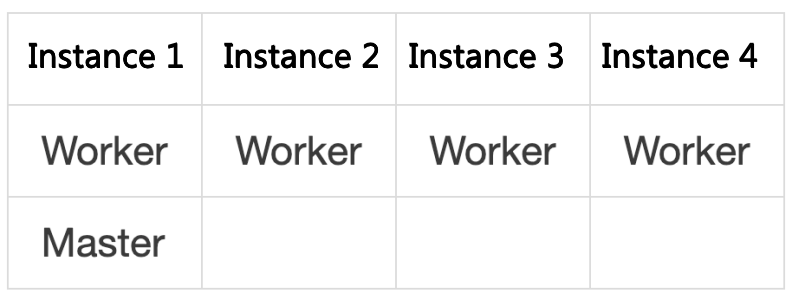#### 2.2.2 Giraph

• System Version: 1.3.0

• Spark version: 2.7.6

Giraph runs jobs based on MapReduce. Deploy each instance as the following figure shows (see Appendix 5.2.1 for Hadoop configuration parameters).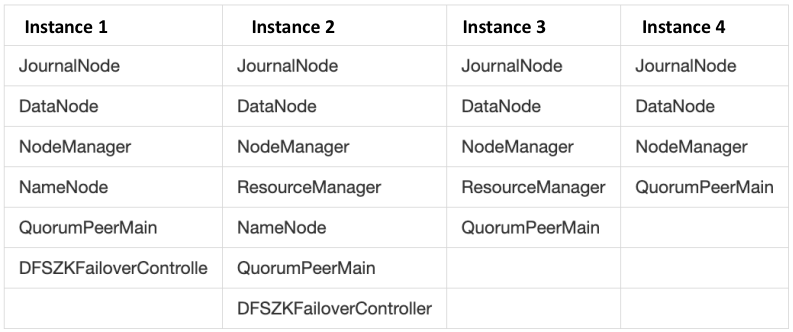#### 2.2.3 Plato

• System Version: 0.1.1

Plato starts 1 process for each instance via Hydra before algorithms are executed.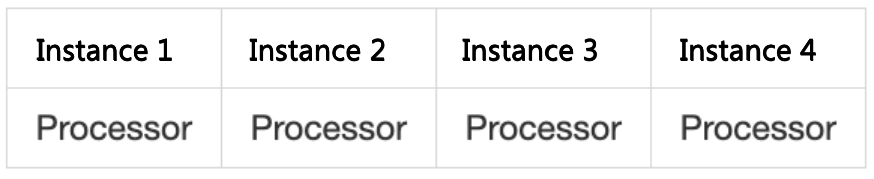### 2.3 Benchmarking Datasets

We use the following 2 graph datasets of different sizes for benchmarking:

• Directedness of graphs: Directed graphs

• Number of vertices: 41,652,230

• Number of edges: 1,468,365,182

clueweb-12

• Directedness of graphs: directed graphs

• Number of vertices: 955,207,488

• Number of edges: 42,574,107,469

### 2.4 Benchmarking Algorithms

We selected 3 typical graph algorithms among the Graphalytics benchmarking algorithms proposed by the LDBC (Linked Data Benchmarking Committee) for benchmarking: PageRank, connected-component, and SSSP.

To ensure the fairness of benchmarking, we unified the algorithm operation parameters of each framework and rewrote the algorithm implementation code of some frameworks to ensure the equivalence of the algorithm operation results of each framework (refer to Appendix 5.1, Appendix 5.2, and Appendix 5.3 for the detailed configuration parameters and source code implementation of each framework).

#### 2.4.1 PageRank

PageRank is a centrality measure for nodes in a graph. It is used to measure the importance of a node in the graph.

Approach: PageRank is a full-space iterative algorithm. Each vertex has an initial rank value, which is taken as the importance of the vertex. For each iteration, the rank value of each vertex updates. The new rank value of a vertex in one iteration is calculated by the rank value of its neighbors in the previous iteration. The new rank value of that vertex can continue to "contribute" to the vertices that it points to in the next iteration. The algorithm terminates when the number of iterations reaches the specified value, or when the change in the rank value of all vertices in the graph is less than a specified threshold.

The unified algorithm operation parameters:

• Number of iterations: 100
• Terminating threshold: 0

#### 2.4.2 Connected Component

The connected-component algorithm is used to detect and count the number of connected subgraphs in a disconnected graph. This benchmarking uses a one-way connected graph algorithm for directed graphs.

Approach: The connected-component algorithm is a non-full-space iterative algorithm. We use labels to represent the connected subgraphs to which the vertices belong. At the beginning of the algorithm, the label value of each vertex is initialized to an id and is set to the active state. In iterations, the vertex in the active state sends its label value to its neighboring vertices, and the neighboring vertices determine that if the received label value is smaller than their own, they update their labels to the smaller value and set themselves to the active state. The algorithm terminates when there are no vertices in the graph with an active state, i.e., no messages are passed. Vertices with the same label value are classified in the same connected subgraph.

#### 2.4.3 Single-Source Shortest Path

Single-Source Shortest Path, also known as SSSP, is a graph algorithm that calculates the shortest path from a source vertex to all other vertices in the graph.

Approach: SSSP is also a non-full-space iterative algorithm. We use `dist` to stand for the shortest distance from a vertex to the source vertex. At the beginning of the algorithm, the `dist` value for the source vertex is initialized to `0` and the `dist` values for other vertices are set to infinity, and the source vertex is set to the active state. In iterations, the vertex in the active state sends its `dist` value to its neighboring vertices, and the neighboring vertices determine that if the received `dist` value (`dist` + 1) is smaller than their own, they update their `dist` values to the smaller value and set themselves to the active state. The algorithm terminates when there are no vertices in the graph with an active state, i.e., no messages are passed.

The unified algorithm operation parameters:

• Source vertex `id`: 0

## 3. Benchmarking Results

We ran three algorithms (PageRank, connected-component, SSSP) with GraphX, Giraph, and Plato in single-node (1 node), two-node (2 nodes), and four-node (4 nodes) deployment modes respectively, and counted the time consumption and peak memory usage of each.

The results of two datasets are presented below (see Appendix 5.4 for details.)

#### 3.1.1 Results

Note: Graphx is not able to run algorithms within 10 hours on 1 node, so the results of Graphx on 1 node are not shown.

PageRank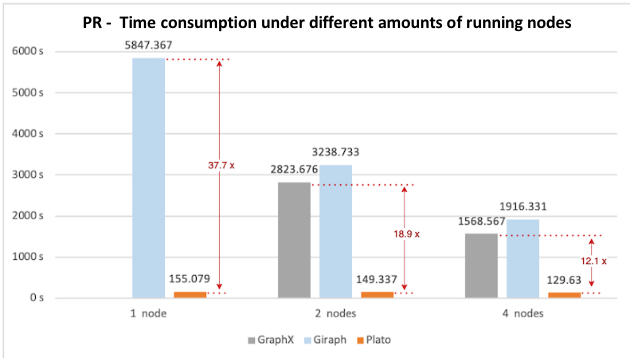Figure 1. PageRank benchmarking results of time consumption with different numbers of running nodes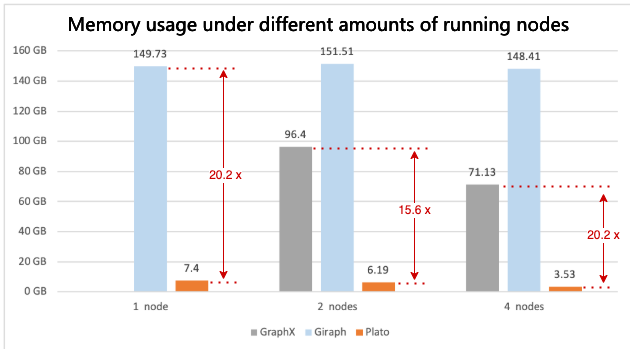Figure 2. PageRank benchmarking results of peak memory usage with different numbers of running nodes

Connected Component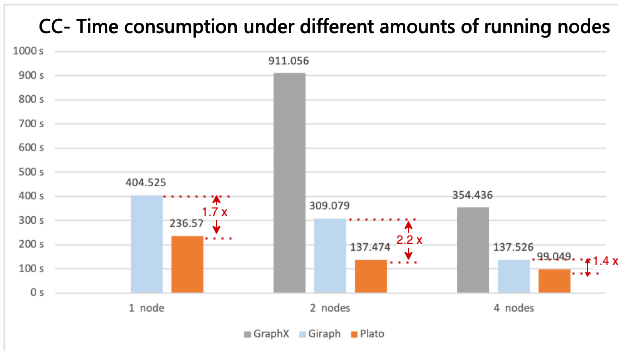Figure 3. Connected Component benchmarking results of time consumption with different numbers of running nodes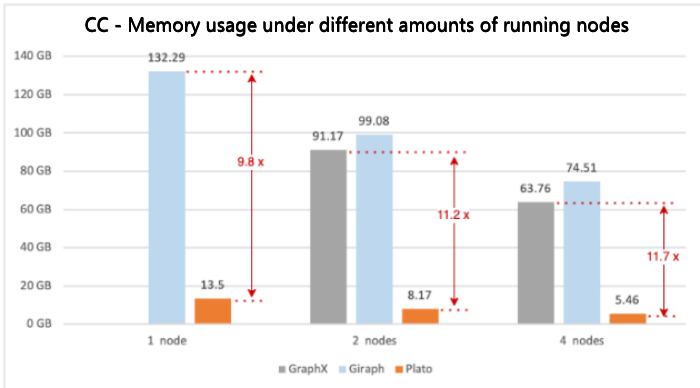Figure 4. Connected Component benchmarking results of peak memory usage with different numbers of running nodes

SSSP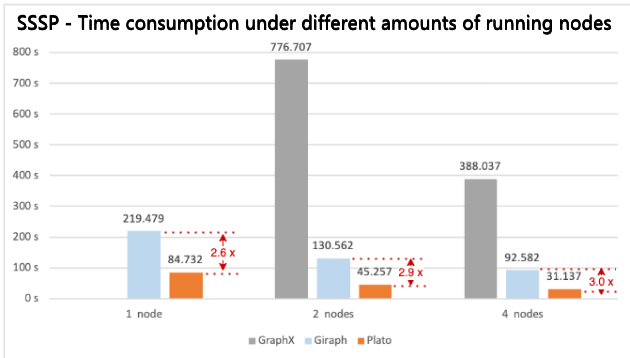Figure 5. SSSP benchmarking results of time consumption with different numbers of running nodes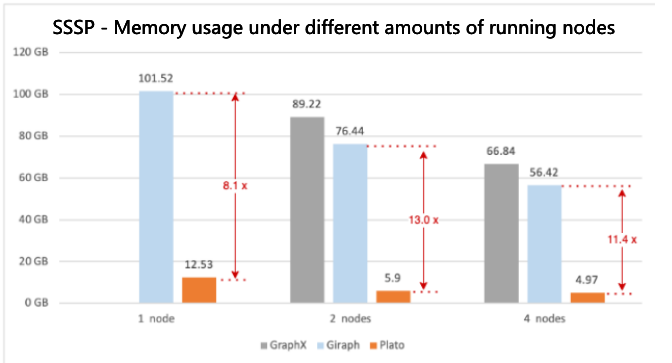Figure 6. SSSP benchmarking results of peak memory usage with different numbers of running nodes

#### 3.1.2 Analysis

• GraphX: The time consumption and peak memory usage results of running those algorithms are both high. Due to the invariance of the underlying data model RDDs, a large number of new RDDs are generated as intermediate results during the computing process. Although GraphX optimizes the reuse of invariant vertices and edges to a certain extent, the framework limitation still leads to large memory consumption and poor performance. In particular, in the single-node scenario, these algorithms cannot be executed within 10 hours.

• Giraph: The overall performance and memory overhead are comparable to GraphX. Giraph stores graph data based on map containers which results in high memory usage. The "long tail" problem slows down the overall running time of the algorithm. Part of the reason is the high cost due to the direct communication between vertices. Especially when there are super nodes, the communication cost and message cache usage are high.

• Plato: It cuts graphs and divides vertices into partitions. Each vertex and all its outgoing and incoming edges are in the same partition. It ensures efficient execution in Pull and Push communication modes.

Figure 7 shows the cut for the Pull communication mode, where one vertex and its incoming and outgoing edges are divided into one partition. Due to the vertex being partitioned, the vertex may be stored multiple times, i.e. a vertex may have multiple mirror vertices (yellow circles in the figure), but only 1 master vertex (white circle). The data of the vertex is stored on the master vertex, and the mirror vertices act as a "bridge" for message passing.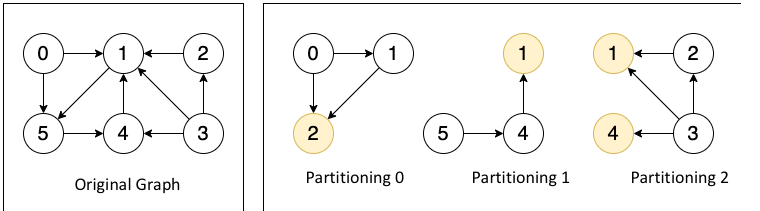Figure 7. Cut for the Pull communication mode

PageRank uses Pull communication mode because it is a full-space iterative algorithm. A complete Pull communication process is divided into two phases, SIGNAL, and SLOT.

As shown in Figure 8, take the example of updating the rank value of vertex 1 (only simulating the computing process). In the SIGNAL phase, vertex 1 in all partitions (master and mirror vertices) collects the rank values from the incoming neighbors and aggregates them locally, and the aggregated rank values are sent to partition 0 where the master vertex 1 is located; In the SLOT phase, the master vertex in the partition 0 performs the final merge of the rank values and gets a new rank value. It can be seen that in this mode, to complete the message passing of one vertex, only (number of partitions - 1) messages need to be sent at most, which significantly reduces the amount of inter-process communications and improves the performance.

Figure 1 shows that Plato performs the PageRank algorithm much faster than GraphX / Giraph. For both connected-component and SSSP, Plato uses an adaptive switching dual communication mode that selects the communication mode with better performance based on the ratio of the number of active edges. From figure 3 and figure 5, compared with the other two frameworks, Plato's performance is one order of magnitude better. In terms of graph storage, Plato greatly reduces memory usage through a well-designed data structure. Moreover, its vertex index and edge array structure are designed to achieve an o(1) time overhead for fetching neighbors. In conclusion, Plato is one order of magnitude faster than GraphX / Giraph in terms of computing speed and one order of magnitude smaller in terms of memory requirement.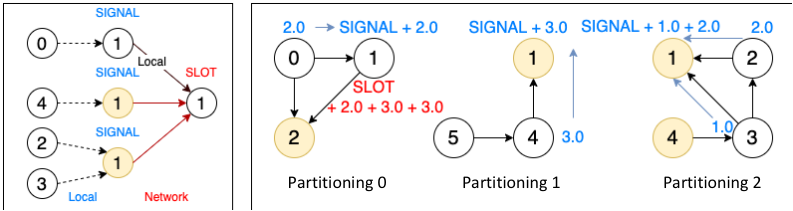### 3.2 Large-Size Dataset clueweb-12

Due to machine resource constraints, we only benchmark these frameworks in the 4 nodes scenario for clueweb-12, a very large dataset with one billion vertices and ten billion edges.

#### 3.2.1 Results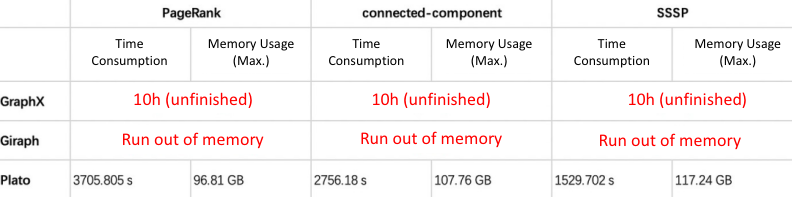#### 3.2.2 Analysis

• GraphX / Giraph: As explained in Section 3.1.2, the memory usage and performance of GraphX / Giraph are not able to complete algorithms within 10 hours on 4 nodes. With this very large dataset and four-node resources, both frameworks are unavailable.

• Plato: Because of the excellent design of the communication model and the sophisticated implementation of the storage structure (see Section 3.1.2), Plato can run these algorithms in a four-node deployment mode with a maximum execution time of fewer than 70 minutes, even with very large datasets, and the memory usage of a single machine is far from the memory limit (192 GB).

## 4. Conclusion

We have the following conclusions after summarizing the results of the three algorithms:

• GraphX has unsatisfactory execution efficiency and memory usage due to the invariance of the underlying RDD.

• Giraph's map container-based graph data structure leads to high memory consumption, and the native vertex-to-vertex communication mode also results in low performance.

• Plato's block partitioning structure and dual engine communication modes, and optimized underlying storage structure design make it far better than the other two frameworks in terms of execution efficiency and memory consumption.

Based on the above conclusions, Plato is a better choice for large-scale graph computing.

## 5. Appendix

### 5.1 GraphX Configurations and the Source Code

spark-defaults.conf

``````spark.driver.cores 2
spark.driver.memory 2g
spark.executor.memory 128g
spark.local.dir /opt/meituan/appdatas/spark-tmp
``````

#### 5.1.2 Execution Script and the Source Code

PageRank execution script

``````/opt/meituan/appdatas/spark-3.1.2-bin-hadoop3.2/bin/spark-submit \ # A bin file for submitting spark jobs.
--deploy-mode cluster \ # Cluster deployment
--master spark://HOST:PORT \ # Specifying the master node's address.
--class PageRankDemo \ # Algorithm execution class
/opt/meituan/appdatas/graphx-runtime/graphx-spark.jar \ # Algorithm execution JAR package
spark://HOST:PORT \ # Specifying the master node's address.
"/opt/meituan/appdatas/graphx-runtime/twitter-2010-s/*" \ # Inputting the file or folder name (will scan all CSV files within.)
100 # The max iteration number
``````

PageRank execution class: PageRankDemo.scala（ custom implementation）

``````import java.io.File
import org.apache.spark.graphx.{Edge, Graph}
import org.apache.spark.rdd.RDD
import org.apache.spark.{SparkConf, SparkContext}
object PageRankDemo {
def main(args: Array[String]): Unit = {
val master = if (args.length > 0) args(0) else "local[*]"
val input = if (args.length > 1) args(1) else "test.csv"
val maxIter = if (args.length > 2) args(2).toInt else 10
var watchTs = System.currentTimeMillis()
val conf = new SparkConf()
.setAppName("Spark PageRank")
.setMaster(master)
.set("spark.ui.port", "8415")
val spark = new SparkContext(conf)
val edges = links.map( line => line.split(",") )
.map( line => ( line(0).toLong, line(1).toLong) )
val graph = Graph.fromEdgeTuples(edges, 1)
println("[PERF] load edges: " + links.count() + ", init graph cost: " + (System.currentTimeMillis() - watchTs))
watchTs = System.currentTimeMillis()
val ranks = graph.staticPageRank(maxIter).vertices
println("[PERF] rank cost: " + (System.currentTimeMillis() - watchTs))
watchTs = System.currentTimeMillis()
val outputPath = "/tmp/graphx_pr_out_csv"
Util deleteDir(new File(outputPath))
ranks.saveAsTextFile(outputPath)
println("[PERF] save output cost: " + (System.currentTimeMillis() - watchTs))
}
}
``````

connected-component execution script

``````/opt/meituan/appdatas/spark-3.1.2-bin-hadoop3.2/bin/spark-submit \ # A bin file for submitting Spark jobs.
--deploy-mode cluster \ # Cluster deployment
--master spark://HOST:PORT \ # Specifying the master node's address.
--class ConnectedComponentsDemo \ # Execution class
/opt/meituan/appdatas/graphx-runtime/graphx-spark.jar \ # Execution JAR package
spark://HOST:PORT \ # Specifying the master node's address.
"/opt/meituan/appdatas/graphx-runtime/twitter-2010-s/*" \ # Inputting the file or folder name (will scan all CSV files within).
``````

connected-component execution class: ConnectedComponentsDemo.scala（Custom implementation）

``````import java.io.File
import org.apache.spark.graphx.{Edge, Graph}
import org.apache.spark.rdd.RDD
import org.apache.spark.{SparkConf, SparkContext}
object ConnectedComponentsDemo {
def main(args: Array[String]): Unit = {
val master = if (args.length > 0) args(0) else "local[*]"
val input = if (args.length > 1) args(1) else "test.csv"
var watchTs = System.currentTimeMillis()
val conf = new SparkConf()
.setAppName("Spark ConnectedComponents")
.setMaster(master)
.set("spark.ui.port", "8415")
val spark = new SparkContext(conf)
val edges = links.map( line => line.split(",") )
.map( line => ( line(0).toLong, line(1).toLong) )
val graph = Graph.fromEdgeTuples(edges, 1)
println("[PERF] load edges: " + links.count() + ", init graph cost: " + (System.currentTimeMillis() - watchTs))
watchTs = System.currentTimeMillis()
val ranks = ConnectedComponentsNew.run(graph).vertices
println("[PERF] rank cost: " + (System.currentTimeMillis() - watchTs))
watchTs = System.currentTimeMillis()
val outputPath = "/tmp/graphx_cc_out_csv"
Util deleteDir(new File(outputPath))
ranks.saveAsTextFile(outputPath)
println("[PERF] save output cost: " + (System.currentTimeMillis() - watchTs))
}
}
``````

`

SSSP execution script

``````/opt/meituan/appdatas/spark-3.1.2-bin-hadoop3.2/bin/spark-submit \ # A bin file for submitting Spark jobs.
--deploy-mode cluster \ # Cluster deployment
--master spark://HOST:PORT \ # Specifying the master node's address.
--class SsspDemo \ # Execution class
/opt/meituan/appdatas/graphx-runtime/graphx-spark.jar \ # Execution JAR package
spark://HOST:PORT \ # Specifying the master node's address.
"/opt/meituan/appdatas/graphx-runtime/twitter-2010-s/*" \ # Inputting the file or folder name (will scan all CSV files within).
0 # Specifying the source vertex.
``````

SSSP execution: SsspDemo.scala（custom implementation）

``````import java.io.File
import org.apache.spark.graphx.{Edge, EdgeDirection, Graph, VertexId}
import org.apache.spark.rdd.RDD
import org.apache.spark.{SparkConf, SparkContext}
object SsspDemo {
def main(args: Array[String]): Unit = {
val master = if (args.length > 0) args(0) else "local[*]"
val input = if (args.length > 1) args(1) else "test.csv"
val sourceId = if (args.length > 2) args(2).toInt else 0
val maxIter = if (args.length > 3) args(3).toInt else Int.MaxValue
var watchTs = System.currentTimeMillis()
val conf = new SparkConf()
.setAppName("Spark SSSP")
.setMaster(master)
.set("spark.ui.port", "8415")
val spark = new SparkContext(conf)
.map( line => line.split(",") )
.map( line => Edge(line(0).toLong, line(1).toLong, 1.0d))
val vertexes: RDD[(VertexId, Double)] = edges
.flatMap(edge => Array(edge.srcId, edge.dstId))
.distinct()
.map(id =>
if (id == sourceId) (id, 0.0)
else (id, Double.PositiveInfinity)
)
val defaultVertex = -1.0d
val initialGraph: Graph[(Double), Double] = Graph(vertexes, edges, defaultVertex)
println("[PERF] load edges: " + links.count() + ", init graph cost: " + (System.currentTimeMillis() - watchTs))
watchTs = System.currentTimeMillis()
val sssp = initialGraph.pregel(Double.PositiveInfinity, maxIter, EdgeDirection.Out)(
//Vertex Program
(id, dist, newDist) => {
if (dist <= newDist) dist else newDist
},
//Send Message
triplet => {
if (triplet.srcAttr + triplet.attr < triplet.dstAttr) {
Iterator((triplet.dstId, triplet.srcAttr + triplet.attr))
} else {
Iterator.empty
}
},
//Merge Message
(a, b) => {
math.min(a, b)
}
)
println("[PERF] rank cost: " + (System.currentTimeMillis() - watchTs))
watchTs = System.currentTimeMillis()
val outputPath = "/tmp/graphx_sssp_out_csv"
Util deleteDir(new File(outputPath))
sssp.vertices.saveAsTextFile(outputPath)
println("[PERF] save output cost: " + (System.currentTimeMillis() - watchTs))
}
}
``````

### 5.2 Giraph Configurations and the Source Code

yarn-site.xml

``````<configuration>

<!-- Site specific YARN configuration properties -->
<property>
<name>yarn.nodemanager.aux-services</name>
<value>mapreduce_shuffle</value>
</property>
<property>
<name>yarn.resourcemanager.ha.enabled</name>
<value>true</value>
</property>
<property>
<name>yarn.resourcemanager.cluster-id</name>
<value>rmCluster</value>
</property>
<property>
<name>yarn.resourcemanager.ha.rm-ids</name>
<value>rm1,rm2</value>
</property>
<property>
<name>yarn.resourcemanager.hostname.rm1</name>
<value>ip2</value>
</property>
<property>
<name>yarn.resourcemanager.hostname.rm2</name>
<value>ip3</value>
</property>
<property>
<value>ip1:2181,ip2:2181,ip3:2181,ip4:2181</value>
</property>
<property>
<name>yarn.resourcemanager.recovery.enabled</name>
<value>true</value>
</property>
<property>
<name>yarn.resourcemanager.store.class</name>
</property>
<property>
<value>ip2:8088</value>
</property>
<property>
<value>ip3:8088</value>
</property>
<property>
<name>yarn.application.classpath</name>
<value>
</value>
</property>
<property>
<name>yarn.scheduler.minimum-allocation-mb</name>
<value>512</value>
</property>
<property>
<name>yarn.scheduler.maximum-allocation-mb</name>
<value>185344</value>
</property>
<property>
<name>yarn.nodemanager.resource.memory-mb</name>
<value>185344</value>
</property>
<property>
<name>yarn.app.mapreduce.am.resource.mb</name>
<value>4096</value>
</property>
<property>
<name>yarn.app.mapreduce.am.command-opts</name>
<value>-Xmx3276m</value>
</property>
<property>
<name>yarn.nodemanager.resource.cpu-vcores</name>
<value>48</value>
</property>
<property>
<name>yarn.scheduler.maximum-allocation-vcores</name>
<value>192</value>
</property>
<property>
<value>8192</value>
</property>
</configuration>
``````

Based on debugging, the execution performance is optimal when the PageRank algorithm starts 39 map tasks per machine, and the connected-component and SSSP algorithms start 19 map tasks per machine (only as a reference setting for the same machine configurations).

``````<configuration>
<property>
<name>mapreduce.framework.name</name>
<value>yarn</value>
</property>
<property>
<value>ip1.mt:10020</value>
</property>
<property>
<value>ip1:19888</value>
</property>
<property>
<name>mapreduce.jobhistory.joblist.cache.size</name>
<value>20000</value>
</property>
<property>
<name>mapreduce.jobhistory.done-dir</name>
<value>\${yarn.app.mapreduce.am.staging-dir}/history/done</value>
</property>
<property>
<name>mapreduce.jobhistory.intermediate-done-dir</name>
<value>\${yarn.app.mapreduce.am.staging-dir}/history/done_intermediate</value>
</property>
<property>
<name>yarn.app.mapreduce.am.staging-dir</name>
</property>
<property>
<name>yarn.app.mapreduce.am.env</name>
</property>
<property>
<name>mapreduce.map.env</name>
</property>
<property>
<name>mapreduce.reduce.env</name>
</property>
<property>
<name>mapred.job.tracker</name>
<value>ip1.mt:54311</value>
</property>
<property>
<value>40</value>
</property>
<property>
<value>2</value>
</property>
<property>
<name>mapreduce.map.memory.mb</name>
<value>4608</value>
</property>
<property>
<name>mapreduce.reduce.memory.mb</name>
<value>10</value>
</property>
<property>
<name>mapreduce.map.java.opts</name>
<value>-Xmx4147m</value>
</property>
<property>
<name>mapreduce.reduce.java.opts</name>
<value>-Xmx8m</value>
</property>
<property>
<value>36000000</value>
</property>
<property>
<name>mapreduce.job.counters.limit</name>
<value>500</value>
</property>
</configuration>

<configuration>
<property>
<name>mapreduce.framework.name</name>
<value>yarn</value>
</property>
<property>
<value>ip1:10020</value>
</property>
<property>
<value>ip1:19888</value>
</property>
<property>
<name>mapreduce.jobhistory.joblist.cache.size</name>
<value>20000</value>
</property>
<property>
<name>mapreduce.jobhistory.done-dir</name>
<value>\${yarn.app.mapreduce.am.staging-dir}/history/done</value>
</property>
<property>
<name>mapreduce.jobhistory.intermediate-done-dir</name>
<value>\${yarn.app.mapreduce.am.staging-dir}/history/done_intermediate</value>
</property>
<property>
<name>yarn.app.mapreduce.am.staging-dir</name>
</property>
<property>
<name>yarn.app.mapreduce.am.env</name>
</property>
<property>
<name>mapreduce.map.env</name>
</property>
<property>
<name>mapreduce.reduce.env</name>
</property>
<property>
<name>mapred.job.tracker</name>
<value>xr-nlpkg-graph-proxy01.mt:54311</value>
</property>
<property>
<value>20</value>
</property>
<property>
<value>2</value>
</property>
<property>
<name>mapreduce.map.memory.mb</name>
<value>9216</value>
</property>
<property>
<name>mapreduce.reduce.memory.mb</name>
<value>10</value>
</property>
<property>
<name>mapreduce.map.java.opts</name>
<value>-Xmx8294m</value>
</property>
<property>
<name>mapreduce.reduce.java.opts</name>
<value>-Xmx8m</value>
</property>
<property>
<value>36000000</value>
</property>
<property>
<name>mapreduce.job.counters.limit</name>
<value>500</value>
</property>
</configuration>
``````
##### 5.2.2 Execution Scritp and the Source Code

Parsing class for edge input files: LongStaticDoubleTextEdgeInputFormat.java（Custom implementation）

``````package org.apache.giraph.io.formats;
import org.apache.giraph.utils.IntPair;
import java.io.IOException;
import java.util.regex.Pattern;
/**
* Simple text-based {@link org.apache.giraph.io.EdgeInputFormat} for
* unweighted graphs with int ids.
*
* Each line consists of: source_vertex, target_vertex
*/
public class LongStaticDoubleTextEdgeInputFormat extends
TextEdgeInputFormat<LongWritable, FloatWritable> {
/** Splitter for endpoints */
private static final Pattern SEPARATOR = Pattern.compile(",");
@Override
InputSplit split, TaskAttemptContext context) throws IOException {
}
@Override
protected IntPair preprocessLine(Text line) throws IOException {
String[] tokens = SEPARATOR.split(line.toString());
return new IntPair(Integer.parseInt(tokens),
Integer.parseInt(tokens));
}
@Override
protected LongWritable getSourceVertexId(IntPair endpoints)
throws IOException {
return new LongWritable(endpoints.getFirst());
}
@Override
protected LongWritable getTargetVertexId(IntPair endpoints)
throws IOException {
return new LongWritable(endpoints.getSecond());
}
@Override
protected FloatWritable getValue(IntPair endpoints) throws IOException {
// This class only handles unweighted graphs. The edge weights are initialized to the default value of 1.0.
return new FloatWritable(1.0f);
}
}
}
``````

PageRank execution script

``````hadoop fs -rm -r /giraph-out-pr
org.apache.giraph.GiraphRunner \ # Giraph launch class
org.apache.giraph.examples.SimplePageRankComputation \ # Algorithm execution class
-mc org.apache.giraph.examples.SimplePageRankComputation\\$SimplePageRankMasterCompute \ # The main class of the algorithm (special configuratio for PageRank)
-eif org.apache.giraph.io.formats.LongStaticDoubleTextEdgeInputFormat \ # Parsing class for inputting edge files (Custom implementation)
-eip /giraph-input/twitter-2010-s \ # File or folder name for edge input files, where all CSV files are auto scanned).
-vof org.apache.giraph.io.formats.IdWithValueTextOutputFormat \ # Output class for vertex data
-op /giraph-out-pr \ # Output path for vertex data
-w 39*N \ # The number of all launched tasks (N is the number of machines)
-ca giraph.SplitMasterWorker=true # Specifies the customized parameter
``````

connected-component execution script

``````hadoop fs -rm -r /giraph-out-cc
org.apache.giraph.GiraphRunner \ # Giraph launch class
org.apache.giraph.examples.ConnectedComponentsComputation \ # Algorithm execution class
-eif org.apache.giraph.io.formats.LongStaticDoubleTextEdgeInputFormat \ # Parsing class for inputting edge files (Custom implementation)
-eip /giraph-input/twitter-2010-s \ # File or folder name for edge input files, where all CSV files are auto scanned).
-vof org.apache.giraph.io.formats.IdWithValueTextOutputFormat \ # Output path for vertex data
-op /giraph-out-cc \ # Output path for vertex data
-w 19*N \ # The number of all launched tasks (N is the number of machines)
-ca giraph.SplitMasterWorker=true # Specifies the customized parameter
``````

connected-component execution class: ConnectedComponentsComputation.java (rewrote the logic)

``````package org.apache.giraph.examples;
import org.apache.giraph.graph.BasicComputation;
import org.apache.giraph.edge.Edge;
import org.apache.giraph.graph.Vertex;
import java.io.IOException;
@Algorithm(
name = "Connected components",
description = "Finds connected components of the graph"
)
public class ConnectedComponentsComputation extends
BasicComputation<LongWritable, LongWritable, FloatWritable, LongWritable> {
/**
* Propagates the smallest vertex id to all neighbors. Will always choose to
* halt and only reactivate if a smaller id has been sent to it.
*
* @param vertex Vertex
* @param messages Iterator of messages from the previous superstep.
* @throws IOException
*/
@Override
public void compute(
Vertex<LongWritable, LongWritable, FloatWritable> vertex,
Iterable<LongWritable> messages) throws IOException {
if (getSuperstep() == 0 ||
getSuperstep() == 1 && vertex.getNumEdges() == 0) {
// The initialized lable value is the ID of the vertex.
vertex.setValue(vertex.getId());
}
long currentComponent = vertex.getValue().get();
if (getSuperstep() == 0) {
for (Edge<LongWritable, FloatWritable> edge : vertex.getEdges()) {
LongWritable neighbor = edge.getTargetVertexId();
sendMessage(neighbor, vertex.getValue());
}
vertex.voteToHalt();
return;
}
boolean changed = false;
for (LongWritable message : messages) {
long candidateComponent = message.get();
if (candidateComponent < currentComponent) {
currentComponent = candidateComponent;
changed = true;
}
}
// propagate new component id to the neighbors
if (changed) {
vertex.setValue(new LongWritable(currentComponent));
sendMessageToAllEdges(vertex, vertex.getValue());
}
vertex.voteToHalt();
}
}
``````

SSSP execution script

``````hadoop fs -rm -r /giraph-out-sssp
org.apache.giraph.GiraphRunner \ # Giraph launch class
org.apache.giraph.examples.SimpleShortestPathsComputation \ # Algorithm execution class
-eif org.apache.giraph.io.formats.LongStaticDoubleTextEdgeInputFormat \ # Parsing class for inputting edge files (Custom implementation)
-eip /giraph-input/twitter-2010-s \ # File or folder name for edge input files, where all CSV files are auto scanned).
-vof org.apache.giraph.io.formats.IdWithValueTextOutputFormat \ # Output path for vertex data
-op /giraph-out-sssp \ # Output path for vertex data
-w 19*N \ # The number of all launched tasks (N is the number of machines)
-ca giraph.SplitMasterWorker=true,SimpleShortestPathsVertex.sourceId=0 # Specifies the customized parameter
``````

SSSP execution class: SimpleShortestPathsComputation.java（rewrote the logic）

``````package org.apache.giraph.examples;
import org.apache.giraph.graph.BasicComputation;
import org.apache.giraph.conf.LongConfOption;
import org.apache.giraph.edge.Edge;
import org.apache.giraph.graph.Vertex;
import org.apache.log4j.Logger;
import java.io.IOException;
/**
* Demonstrates the basic Pregel shortest paths implementation.
*/
@Algorithm(
name = "Shortest paths",
description = "Finds all shortest paths from a selected vertex"
)
public class SimpleShortestPathsComputation extends BasicComputation<
LongWritable, DoubleWritable, FloatWritable, DoubleWritable> {
/** The shortest paths id */
public static final LongConfOption SOURCE_ID =
new LongConfOption("SimpleShortestPathsVertex.sourceId", 1,
"The shortest paths id");
/** Class logger */
private static final Logger LOG =
Logger.getLogger(SimpleShortestPathsComputation.class);
/**
* Is this vertex the source id?
*
* @param vertex Vertex
* @return True if the source id
*/
private boolean isSource(Vertex<LongWritable, ?, ?> vertex) {
return vertex.getId().get() == SOURCE_ID.get(getConf());
}
@Override
public void compute(
Vertex<LongWritable, DoubleWritable, FloatWritable> vertex,
Iterable<DoubleWritable> messages) throws IOException {
if (getSuperstep() == 0 ||
!isSource(vertex) && vertex.getValue().get() == 0.0) {
// The distance value of all vertices are initialized to the max.
vertex.setValue(new DoubleWritable(Double.MAX_VALUE));
}
double minDist = isSource(vertex) ? 0d : Double.MAX_VALUE;
for (DoubleWritable message : messages) {
minDist = Math.min(minDist, message.get());
}
if (minDist < vertex.getValue().get()) {
vertex.setValue(new DoubleWritable(minDist));
for (Edge<LongWritable, FloatWritable> edge : vertex.getEdges()) {
double distance = minDist + edge.getValue().get();
sendMessage(edge.getTargetVertexId(), new DoubleWritable(distance));
}
}
vertex.voteToHalt();
}
}
``````

### 5.3 Plato Configurations and the Source Code

#### 5.3.1 Execution Scripts and the Code

PageRank execution script

``````#!/bin/bash
set -ex
ROOT_DIR="/opt/meituan/appdatas/plato-mt-0.1.1" # The path of the source code
WORK_DIR="/opt/meituan/appdatas/plato-runtime" # Working path
MAIN="\$ROOT_DIR/bazel-bin/example/pagerank" # Address of the compiled algorithm binary file
WNUM=N   # Number of process partitions
WCORES=48 # The number of threads in use
MPI_HOSTS="HOST1,HOST2..." # Process IP list (replace with actual machine ip list)
INPUT=\${INPUT:="/opt/meituan/appdatas/graphx-runtime/twitter-2010-s"} # Input file or folder name (all CSV files within are scanned)
OUTPUT=\${OUTPUT:="\$WORK_DIR/../plato-output/pr_output"}            # Output folder name
IS_DIRECTED=\${IS_DIRECTED:=true}   # Directed or undirected graph
EPS=\${EPS:=0}                       # When the delta value is less than this value, iteration ends.
DAMPING=\${DAMPING:=0.85}            # Integer parameter
ITERATIONS=\${ITERATIONS:=100}       # The max iteration number
# param
PARAMS+=" --input \${INPUT} --output \${OUTPUT} --is_directed=\${IS_DIRECTED}"
PARAMS+=" --iterations \${ITERATIONS} --eps \${EPS} --damping \${DAMPING}"
# mpich
MPIRUN_CMD=\${MPIRUN_CMD:="\$ROOT_DIR/3rd/mpich/bin/mpiexec.hydra"}
# test
# run
\${MPIRUN_CMD} -n \${WNUM} -host \$MPI_HOSTS \${MAIN} \${PARAMS} # The host parameter specifies the execution machine, which needs to be accessible via the ssh public key.
``````

connected-component execution script

``````#!/bin/bash
set -ex
ROOT_DIR="/opt/meituan/appdatas/plato-mt-0.1.1" # The path of the source code
WORK_DIR="/opt/meituan/appdatas/plato-runtime" # Working path
MAIN="\$ROOT_DIR/bazel-bin/example/cgm_simple" # Address of the compiled algorithm binary file
WNUM=N   # The number of process partitions(replace N with the number of machines)
WCORES=48 # The number of threads in use
MPI_HOSTS="HOST1,HOST2..." # Process IP list (replace with actual machine ip list)
INPUT=\${INPUT:="/opt/meituan/appdatas/graphx-runtime/twitter-2010-s"} # Input file or folder name (all CSV files within are scanned)
OUTPUT=\${OUTPUT:="\$WORK_DIR/../plato-output/cc_output"}            # Output folder name
IS_DIRECTED=\${IS_DIRECTED:=true}   # Directed or undirected graph
OUTPUT_METHOD=\${OUTPUT_METHOD:="all_vertices"} # Output result format
# param
PARAMS+=" --input \${INPUT} --output \${OUTPUT} --is_directed=\${IS_DIRECTED}"
PARAMS+=" --output_method \${OUTPUT_METHOD}"
# mpich
MPIRUN_CMD=\${MPIRUN_CMD:="\$ROOT_DIR/3rd/mpich/bin/mpiexec.hydra"}
# test
# run
\${MPIRUN_CMD} -n \${WNUM} -host \$MPI_HOSTS \${MAIN} \${PARAMS} # The host parameter specifies the execution machine, which needs to be accessible via the ssh public key.
``````

SSSP execution script

``````#!/bin/bash
set -ex
ROOT_DIR="/opt/meituan/appdatas/plato-mt-0.1.1" # The path of the source code
WORK_DIR="/opt/meituan/appdatas/plato-runtime" # Working path
MAIN="\$ROOT_DIR/bazel-bin/example/sssp_simple" # Address of the compiled algorithm binary file
WNUM=N   # The number of process partitions (replace N with the number of machines)
WCORES=48 # The number of threads in use
MPI_HOSTS="HOST1,HOST2..." # Process IP list (replace with actual machine ip list)
INPUT=\${INPUT:="/opt/meituan/appdatas/graphx-runtime/twitter-2010-s"} # Input file or folder name (all CSV files within are scanned)
OUTPUT=\${OUTPUT:="\$WORK_DIR/../plato-output/sssp_output"}            # Output folder
IS_DIRECTED=\${IS_DIRECTED:=true}   # Directed or undirected graph
# param
PARAMS+=" --input \${INPUT} --output \${OUTPUT} --is_directed=\${IS_DIRECTED}"
# mpich
MPIRUN_CMD=\${MPIRUN_CMD:="\$ROOT_DIR/3rd/mpich/bin/mpiexec.hydra"}
# test
# run
\${MPIRUN_CMD} -n \${WNUM} -host \$MPI_HOSTS \${MAIN} \${PARAMS} # The host parameter specifies the execution machine, which needs to be accessible via the ssh public key.
``````

Plato does not contain SSSP algorithm package, so the algorithm is implemented equivalently.

1. Create `sssp_simple.cc` in the `/example` and add the following content at the end of the Build file.

sssp_simple.cc:

``````#include <cstdint>
#include "glog/logging.h"
#include "gflags/gflags.h"
#include "plato/graph/graph.hpp"
#include "plato/algo/sssp/sssp.hpp"
DEFINE_string(input,       "",     "input file, in csv format, without edge data");
DEFINE_string(output,      "",     "output directory, store the sssp result");
DEFINE_bool(is_directed,   true,   "is graph directed or not");
DEFINE_uint32(root,        0,      "start sssp from which vertex");
DEFINE_int32(alpha,        -1,     "alpha value used in sequence balance partition");
DEFINE_bool(part_by_in,    false,  "partition by in-degree");
bool string_not_empty(const char*, const std::string& value) {
if (0 == value.length()) { return false; }
return true;
}
DEFINE_validator(input, &string_not_empty);
DEFINE_validator(output, &string_not_empty);
void init(int argc, char** argv) {
gflags::ParseCommandLineFlags(&argc, &argv, true);
}
int main(int argc, char** argv) {
plato::stop_watch_t watch;
auto& cluster_info = plato::cluster_info_t::get_instance();
init(argc, argv);
cluster_info.initialize(&argc, &argv);
plato::graph_info_t graph_info(FLAGS_is_directed);
auto graph = plato::create_dualmode_seq_from_path<plato::empty_t>(&graph_info, FLAGS_input,
plato::edge_format_t::CSV, plato::dummy_decoder<plato::empty_t>,
FLAGS_alpha, FLAGS_part_by_in);
plato::algo::sssp_opts_t opts;
opts.root_ = FLAGS_root;
watch.mark("t0");
plato::thread_local_fs_output os(FLAGS_output, (boost::format("%04d_") % cluster_info.partition_id_).str(), true);
auto callback = [&] (plato::vid_t v_i, std::uint32_t value) {
auto& fs_output = os.local();
fs_output << v_i << "," << value << "\n";
};
if (0 == cluster_info.partition_id_) {
LOG(INFO) << "Load graph cost: " << watch.show("load") / 1000.0 << "s";
}
plato::vid_t visited = plato::algo::single_source_shortest_path(graph.second, graph.first,
graph_info, opts, callback);
if (0 == cluster_info.partition_id_) {
LOG(INFO) << "sssp done, visited: " << visited << ", cost: "
<< watch.show("t0") / 1000.0 << "s";
}
return 0;
}
``````

BUILD:

``````cc_binary (
name = "sssp_simple",
srcs = [
"sssp_simple.cc",
],
copts = ['-g', '-O2', ] + PLATO_OPTS,
linkopts = [ ] + PLATO_OPTS,
deps = [
"//3rd/glog:glog",
"//3rd/gflags:gflags",
"//3rd/boost:boost_include",
"//plato/graph:graph",
"//plato/algo/sssp:sssp",
],
defines = [
# "__DCSC_DEBUG__",
],
)
``````
1. Create the `/plato/algo/sssp` directory, and create files `sssp.hpp` and `BUILD` in that directory. sssp.hpp:
``````#ifndef __PLATO_ALGO_SSSP_HPP__
#define __PLATO_ALGO_SSSP_HPP__
#include <cstdint>
#include <cstdlib>
#include "glog/logging.h"
#include "plato/util/perf.hpp"
#include "plato/util/atomic.hpp"
#include "plato/graph/graph.hpp"
#include "plato/engine/dualmode.hpp"
namespace plato { namespace algo {
struct sssp_opts_t {
vid_t root_ = 0;
};
// distance message structures
struct distance_msg_type_t {
vid_t v_i;
std::uint32_t value;
};
/*
* demo implementation of single source shortest path
*
* \tparam INCOMING   graph type, with incoming edges
* \tparam OUTGOING   graph type, with outgoing edges
*
* \param in_edges    incoming edges, dcsc, ...
* \param out_edges   outgoing edges, bcsr, ...
* \param graph_info  base graph-info
* \param opts        sssp options
* \param callback    callback func to ouput result
*
* \return
*    visited vertices count
* */
template <typename INCOMING, typename OUTGOING, typename Callback>
vid_t single_source_shortest_path(
INCOMING& in_edges,
OUTGOING& out_edges,
const graph_info_t& graph_info,
const sssp_opts_t& opts,
Callback&& callback) {
plato::stop_watch_t watch;
auto& cluster_info = plato::cluster_info_t::get_instance();
watch.mark("run");
// Pass in graph data for both cuts to build a dual-mode engine.
dualmode_engine_t<INCOMING, OUTGOING> engine (
std::shared_ptr<INCOMING>(&in_edges,  [](INCOMING*) { }),
std::shared_ptr<OUTGOING>(&out_edges, [](OUTGOING*) { }),
graph_info);
// alloc structs used during bfs
auto visited        = engine.alloc_v_subset(); // Mark the visited vertices
auto active_current = engine.alloc_v_subset(); // Mark the vertices that are active in this iteration
auto active_next    = engine.alloc_v_subset(); // Mark the vertices that are active in the next iteration
auto distance         = engine.template alloc_v_state<std::uint32_t>(); // Store the shortest distance of the vertices
// Initialize the distance of the non-source point to the maximum value and the distance of the source point to 0.
distance.fill(std::numeric_limits<std::uint32_t>::max());
distance[opts.root_] = 0;
// Mark the source as visited
visited.set_bit(opts.root_);
// Mark the source as active
active_current.set_bit(opts.root_);
// Global variable that counts the number of vertices in the active state of all partitions.
plato::vid_t actives = 1;
for (int epoch_i = 0; 0 != actives; ++epoch_i) {
using pull_context_t = plato::template mepa_ag_context_t<distance_msg_type_t>;
using pull_message_t = plato::template mepa_ag_message_t<distance_msg_type_t>;
using push_context_t = plato::template mepa_bc_context_t<distance_msg_type_t>;
watch.mark("t1");
active_next.clear();
actives = engine.template foreach_edges<distance_msg_type_t, plato::vid_t> (
// PUSH
[&](const push_context_t& context, vid_t v_i) {
context.send(distance_msg_type_t {v_i, distance[v_i] + 1});
},
[&](int /*p_i*/, distance_msg_type_t& msg) {
plato::vid_t activated = 0;
auto neighbours = out_edges.neighbours(msg.v_i);
for (auto it = neighbours.begin_; neighbours.end_ != it; ++it) {
plato::vid_t dst = it->neighbour_;
if ((plato::write_min(&distance[dst], msg.value))
) {
active_next.set_bit(dst);
visited.set_bit(dst);
++activated;
}
}
return activated;
},
// PULL
plato::vid_t src = it->neighbour_;
if (active_current.get_bit(src)) {
context.send(pull_message_t {v_i, distance_msg_type_t{v_i, distance[src] + 1}});
break;
}
}
},
[&](int, pull_message_t & msg) {
if (plato::write_min(&distance[msg.v_i_], msg.message_.value)) {
active_next.set_bit(msg.v_i_);
visited.set_bit(msg.v_i_);
return 1;
}
return 0;
},
active_current
);
std::swap(active_current, active_next);
if (0 == cluster_info.partition_id_) {
LOG(INFO) << "active_v[" << epoch_i << "] = " << actives << ", cost: " << watch.show("t1") / 1000.0 << "s";
}
}
if (0 == cluster_info.partition_id_) {
LOG(INFO) << "Run cost: " << watch.show("run") / 1000.0 << "s";
}
watch.mark("output");
// save output
auto active_all = engine.alloc_v_subset();
active_all.fill();
//traverse
auto active_view_all = plato::create_active_v_view(engine.out_edges()->partitioner()->self_v_view(), active_all);
active_view_all.template foreach<vid_t>([&] (vid_t v_i) {
callback(v_i, distance[v_i]);
return 1;
});
if (0 == cluster_info.partition_id_) {
LOG(INFO) << "Output cost: " << watch.show("output") / 1000.0 << "s";
}
visited.sync();
return visited.count();
}
}}  // namespace algo, namespace plato
#endif
BUILD:
cc_library (
name = "sssp",
hdrs = [
"sssp.hpp",
],
srcs = [],
includes = [ ".", ],
deps = [
"//3rd/glog:glog",
"//plato/util:perf",
"//plato/util:atomic",
"//plato/graph:graph",
"//plato/engine:dualmode",
],
defines = [
"__DUALMODE_DEBUG__",
],
copts = [ '-O2', '-Wall', '-std=c++11', ] + PLATO_OPTS,
linkopts = [ ] + PLATO_OPTS,
visibility = ["//visibility:public"],
)
``````

### 5.4 Benchmarking data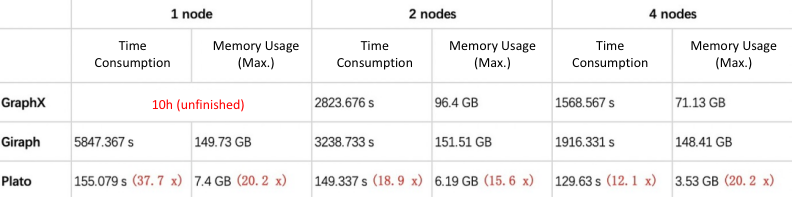`18.9 x`: Represents the runtime ratio of Plato to the better performance one in the other two frameworks in 2 nodes mode (2823.676 / 149.337 = 18.9). That is, in this scenario, Plato performs at least 18.9 times better than the other two frameworks. The same for the other metrics.

connected-component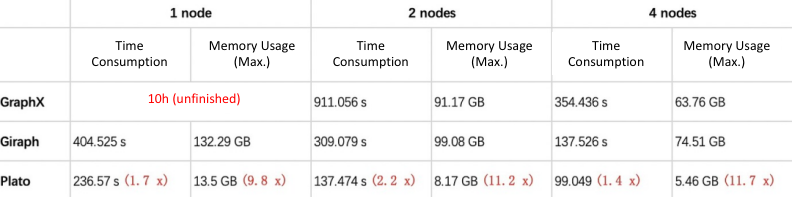SSSP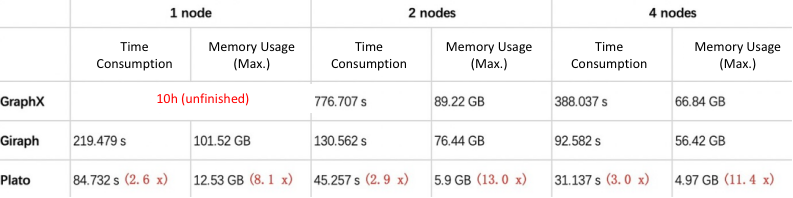5.4.2 clueweb-12 benchmarking data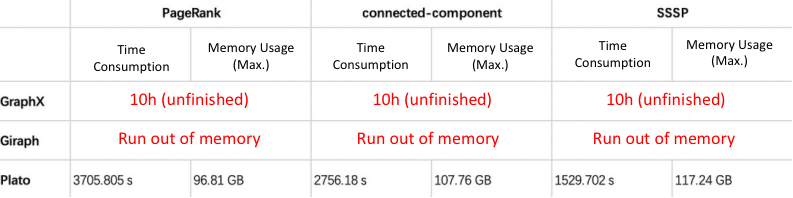## 6. You May Also Like:

Benchmarking the Mainstream Open Source Distributed Graph Databases at Meituan